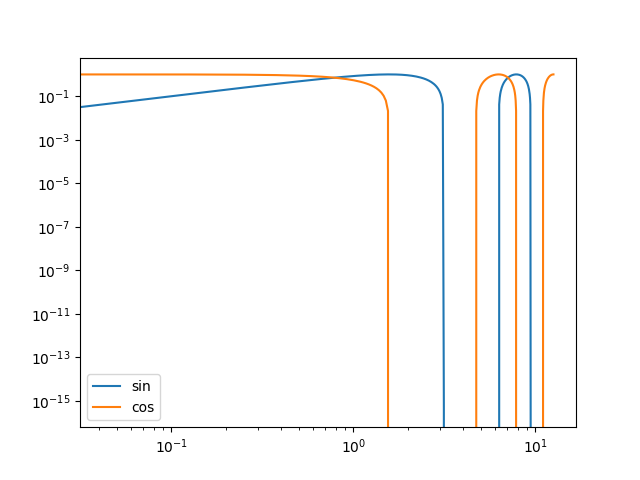# Providing a figure for the thumbnail image#

This example demonstrates how to provide a figure that is displayed as the thumbnail. This is done by specifying the keyword-value pair `sphinx_gallery_thumbnail_path = 'fig path'` as a comment somewhere below the docstring in the example file. In this example, we specify that we wish the figure `demo.png` in the folder `_static` to be used for the thumbnail.

```import numpy as np
import matplotlib.pyplot as plt

# sphinx_gallery_thumbnail_path = '_static/demo.png'
```
```x = np.linspace(0, 4 * np.pi, 301)
y1 = np.sin(x)
y2 = np.cos(x)
```

## Plot 1#

```plt.figure()
plt.plot(x, y1, label="sin")
plt.plot(x, y2, label="cos")
plt.legend()
plt.show()
```## Plot 2#

```plt.figure()
plt.plot(x, y1, label="sin")
plt.plot(x, y2, label="cos")
plt.legend()
plt.xscale("log")
plt.yscale("log")
plt.show()
```Total running time of the script: (0 minutes 2.124 seconds)

Estimated memory usage: 9 MB

Gallery generated by Sphinx-Gallery